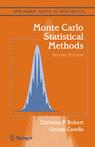## truncated t’s [typo]

Posted in pictures, Statistics with tags , , , , , on March 14, 2014 by xi'an

Last night, I received this email from Piero Foscari (im Hamburg) about my moment derivations for the absolute and the positive t distribution:There might be two typos in the final second moment formula and its derivation (assuming no silly symmetric mistakes in my validation code): the first ν ought to be -ν, and there should be a corresponding scaling factor also for the boundary μ in Pμ,ν-2 since it arises from a change of variable. Btw in the text reference to Fig. 2 |X| wasn’t updated to X+. I hope that this is of some use.

and I checked that indeed I had forgotten the scale factor ν/(ν-2) in the t distribution with ν-2 degrees of freedom as well as the sign… So I modified the note and rearXived it. Sorry about this lack of attention to the derivation!

## Typo in Biometrika 97(3): 747

Posted in Statistics, Travel, University life with tags , , , on November 4, 2010 by xi'an

Yesterday while in Philadelphia airport I got this email from Javier Rubio:

Prof. Robert,

I am a first year PhD student in the University of Warwick. I was reading your paper “Properties of nested sampling” which I found very interesting.  I have a question about it. Is the second equation in pp. 747 correct?
I think this equation is related with the first equation in pp. 12 from your paper “Importance sampling methods for Bayesian discrimination between embedded models”.
Kind regards,
Javier.

Indeed, there is a typo in that this formula (page 747) should be$\widehat{Z}_1=1\bigg/\left\{\dfrac{1}{T}\,\sum_{t=1}^T {g(\theta^{(t)}) }\big/\pi(\theta^{(t)})L(\theta^{(t)})\right\}$

(I checked my LaTeX code and there is a trace of a former \dfrac that got erased but was not replaced with a \big/ symbol… I am quite sorry for the typo, the more because this paper went through many revisions.) There is no typo in the corresponding chapter of Frontiers of Statistical Decision Making and Bayesian Analysis: In Honor of James O. Berger

## Typos…

Posted in Books, R, Statistics with tags , , , on October 6, 2010 by xi'anEdward Kao just sent another typo found both in  Monte Carlo Statistical Methods (Problem 3.21) and in Introducing Monte Carlo Methods with R (Exercise 3.17), namely that$\mathcal{G}a(y,1)$ should be$\mathcal{G}a(1,y).$ I also got another email from Jerry Sin mentioning that matrix summation in the matrix commands of Figure 1.2 of Introducing Monte Carlo Methods with R should be matrix multiplication. And asking for an errata sheet on the webpage of the books, which is clearly necessary and overdue! Here are also a few more typos found by Pierre Jacob and Robin Ryder when working on the translation of Introducing Monte Carlo Methods with R:

## Typo in mixture survey

Posted in Books, Statistics with tags , , , on September 19, 2010 by xi'an

This morning I received the following email

(…) I have a question regarding an algorithm in one of your papers, “Bayesian Modelling and Inference on Mixtures of Distributions“.  On page 33, in the Metropolis-Hastings algorithm for the mixture you accept the proposal if r < u.  As I understand the MH algorithm you accept the proposal with probability r (technically min(r,1)), so I would expect that you accept if u < r.  I cannot see or find a reason elsewhere why r < u works?  If you could clarify why r < u works for the MH algorithm I would really appreciate it. (…)

which rightly points out an embarrassing typo in our mixture survey, published in the Handbook of Statistics, volume 25. Indeed, the inequality should be the reverse,$u, as in the other algorithmic boxes of the survey.

## Typo in Example 3.6

Posted in Books, R, Statistics with tags , , , on September 17, 2010 by xi'anEdward Kao pointed out the following difficulty about Example 3.6 in Chapter 3 of “Introducing Monte Carlo Methods with R”:

I have two questions that have puzzled me for a while. I hope you can shed some lights. They are all about Example 3.6 of your book.

1. On page 74, there is a term x(1-x) for m(x). This is fine. But the term disappeared from (3.5) on p.75. My impression is that this is not a typo. There must be a reason for its disappearance. Can you elaborate?

I am alas afraid this is a plain typo, where I did not report the x(1-x) from one page to the next.

2. On page 75, you have the term “den=dt(normx,3)”. My impression is that you are using univariate t with 3 degrees of freedom to approximate. I thought formally you need to use a bivariatet with 3 degrees of freedom to do the importance sampling. Why would normx=sqrt(x[,1]^2+x[,2]^2) along with a univariate t work?

This is a shortcut that would require more explanation. While the two-dimensional t sample is y, a linear transform of the isotonic x, it is possible to express the density of y via the one-dimensional t density, hence the apparent confusion between univariate and bivariate t densities…